## conference papersJOURNAL OFAPPLIEDCRYSTALLOGRAPHY
ISSN: 1600-5767
Volume 40| Part s1| April 2007| Pages s264-s268

## Salt-concentration dependence of the structure and form factors for the wormlike micelle made from a dual surfactant in aqueous solutions

aThe University of Kitakyushu, Fukuoka 808-0132, Japan, bShiseido Research Center, Polymer Science Research Laboratories, Kanagawa 224-8558, Japan, and cJapan Synchrotron Radiation Research Institute (JASRI/SPring-8), Hyogo 679-5198, Japan
*Correspondence e-mail: sakurai@env.kitakyu-u.ac.jp

(Received 16 August 2006; accepted 10 April 2007; online 21 April 2007)

Small-angle X-ray scattering (SAXS) from dual-surfactant aqueous solutions made from sodium lauryl ether sulfate and coconut fatty acid amido propyl betaine was systematically measured as a function of the net sodium cation concentration, [Na+]*, and the surfactant concentration, CD. The SAXS intensity [I(q)] was normalized to CD and the resultant I(q)/CD was extrapolated to CD = 0 to give a form factor P(q) for each [Na+]* [where q = 4πsin(θ/2)/λ is the magnitude of the scattering vector, λ is the wavelength and 2θ is the scattering angle]. The low-q behaviour of P(q) was consistent with long rigid cylinders. The middle- and high-q profiles fitted well with a core–shell cylinder model for all [Na+]*. The core and total radii (Rc and Rs) did not depend on [Na+]* at all: Rc = 1.2 ± 0.05 and Rs = 3.1 ± 0.05 nm for [Na+]* = 0.42–1.5 mol l−1, indicating that the salt concentration changes did not induce any structural changes and re-assembling of the surfactants comprising the micelles. This fact is in contrast to the rheological behaviour where the relaxation mode strongly depends on [Na+]*. The structure factor [S(q)] was obtained by dividing I(q)/CD by P(q) for each CD and the mean distance (dm) between the micelles was obtained from the first maximum of S(q) versus q plots. The dm value decreased with increasing CD and [Na+]*, which is in good agreement with the theoretical prediction and experimental results for charged wormlike micelle solutions.

### 1. Introduction

Sodium lauryl ether sulfate (LES) and coconut fatty acid amido propyl betaine (AMPB) are the most common ingredients of shampoo [the chemical structures of which are presented in the inset of Fig. 3 of Hiwatari et al. (2004)]. The surfactants are mixed together to tune detergency and other properties for final products. Understanding the rheological properties of the mixed-surfactant aqueous solutions is essential for practical applications and, thus, there have been many studies on this issue (Mu et al., 2002). However, as far as we know, there are only a few studies which focus on the relationship between rheology and micellar structure (Acharya et al., 2006). This paper presents synchrotron small-angle X-ray scattering (SAXS) results for LES/AMPB dual-component aqueous solutions and explores the micellar structures and inter-micellar interactions as a function of the salt and surfactant concentrations.

Being similar to other surfactant aqueous solutions, the LES/AMPB/water system shows viscoelastic behaviour characteristic of micelle solutions. The viscoelasticity strongly depends on the micelle structures and inter-micellar interactions such as entanglement and electrostatic repulsion. Since LES is an anionic surfactant, the micellar structures are mainly determined by the counter cation concentration. Increase of the salt concentration enhances electrostatic shielding effects, which reduce repulsion between the ionic headgroups and thus induce the structural transition of micelles. Generally, the transition is from spherical to cylindrical micelles, and by increasing the cylinder length, the cylindrical micelles start to behave as wormlike chains. When the contour length of the cylinder becomes long enough to form entanglement between the cylinders, the characteristic viscoelasticity emerges (Shikata et al., 1987).

Fig. 1plots the zero-shear viscosity (η) against the net sodium cation concentration, [Na+]*, where the total surfactant concentration (hereinafter denoted by CD and LES:AMPB is fixed at 5:3 by weight ratio) is 8 wt% (203 mmol l−1) (Eguchi & Sakurai, 2007). The viscosity dramatically increases in the [Na+]* range 0–0.75 mol l−1 and showed a broad maximum at around 1.1 mol l−1. Dynamic viscoelastic measurements were carried out at [Na+]* > 0.75 mol l−1. Except for high CD samples, the simple Maxwell model with a single relaxation time (τ) could describe the frequency dependence of the modulus and the plateau modulus as proportional to CD2 (data not shown). These two facts indicate that the origin of the elasticity was the entanglements of the infinitely long wormlike micelles (Ferry, 1980). We found that there are three regions that show different CD dependence on τ. As presented in the inset of Fig. 1, at [Na+]* = 1.0 (except for CD > 0.5 mol l−1), 1.25 and 1.50 mol l−1, τ became independent of CD, indicating that two micelles can cross with each other, the so-called phantom crossing proposed by Shikata et al. (1988). The value of τ decreased with increasing [Na+]*, suggesting that the lifetime of the junction of micelles (tentatively formed when the micelles cross with each other) decreased with increasing [Na+]*. We presumed that the decreasing lifetime of the junction should be related to structural changes of the micelles. At [Na+]* = 0.75 mol l−1 and CD < 0.5 mol l−1, τCD0.5 was seemingly held. This concentration dependence cannot be rationalized by a simple model. At higher CD (> 0.61 mol l−1), for [Na+]* = 0.75 and 1.0 mol l−1, a dramatic increase in τ was observed. As a matter of fact, in this region, both storage and loss moduli could not be fitted with the simple Maxwell model and thus we plotted the longest relaxation time in Fig. 1. The increased relaxation time and deviation from the single relaxation time should be related to the appearance of another relaxation mode, rather than micellar entanglement relaxation. When we prepared samples with larger CD and/or [Na+]* than this region (for example at CD = 0.7 mol l−1 and [Na+]* = 1.0 mol l−1), liquid–liquid phase separation was observed. Therefore, the origin of the slow relaxation is presumably ascribed to composition fluctuation that becomes large near a phase boundary.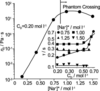Figure 1 [Na+]* dependence of the zero-shear viscosity for the LES/AMPB/water system at CD = 0.20 mol l−1, measured with a B-type viscometer (DV-1+, Brookfield). The inset shows the CD dependence of τ determined with dynamic viscosity measurements for the four [Na+]* concentrations more than 0.75 mol l−1.

As described above, the viscoelastic nature of the present system strongly depends on [Na+]* and apparently should be related to structural changes of the micelles. The aim of this paper is to examine the micellar structures and inter-micellar interactions by comparing with rheological properties.

### 2. Experiments

LES and AMPB were purchased from Toho Chemical Industry Co. Ltd and Sanyo Kasei Industry Co. Ltd, respectively, and used without further purification. The composition ratio of LES:AMPB was always fixed at a 5:3 weight ratio. The counter cation of LES is Na+ and [Na+]* is defined as the total amount of Na+ (added NaCl and the counter Na+). SAXS measurements were carried out at 40B2 SPring-8 using a 1.5 m camera and a Rigaku imaging plate (30 × 30 cm, 1000 × 1000 pixels). The exposure time was 20 min and the relative X-ray intensity and the sample transmittance were determined with ion chambers located in front of or behind the sample. A quartz capillary cell (2 mm diameter, Hilgenberg GmbH) was used for the scattering cell. For all measurements including the solvents we used the same cell. We subtracted the solvent scattering from the sample scattering by use of the following equation: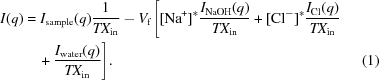q is given by 4πsin(θ/2)/λ, where θ is the scattering angle and λ is the X-ray wavelength. INaOH and IHCl denote the molar scattering from NaOH and HCl solutions, respectively, Iwater is that from water itself, Vf is the volume fraction of the solvent, T is the transmittance and Xin is the read value of the ion chamber in front of the sample when the measurement was carried out.

### 3. Theoretical background and SAXS data analysis

I(q) from isotropic solutions containing cylindrical micelles can be written as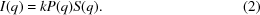Here, k is the averaged number of micelles and proportional to CD when CD is much larger than the critical micelle concentration (CMC); in our case the CMC was 4.8 × 10−3 mol l−1. P(q) and S(q) are the form and structural factors, respectively. For the spherical particles, S(q) can be analytically calculated if an appropriate interparticle interaction potential is given. On the other hand, for randomly oriented rodlike or wormlike micelles, the calculation becomes difficult. Recently, Cannavacciuolo et al. (2002) presented an extensive Monte Carlo study on charged wormlike micelles to calculate S(q). To obtain P(q) from the observed data, I(q)/CD was extrapolated to the limit CD → 0. This is because S(q) = 1 for all q at CD = 0. Once P(q) is obtained, S(q) can be evaluated by dividing I(q)/CD with P(q).

As a matter of fact, this extrapolation does not always work, because the length of the wormlike micelles depends on CD; the length decreases with decreasing CD. However, in our q range, we did not observe any influence from the overall size change. This is because that the overall size has an influence on the form factor in the q range less than approximately q ∼ 1/L, where L is the contour length of the micelle. Viscosity data indicated that the micelle length is long in the all concentrations measured using SAXS. Therefore, the above-mentioned extrapolation should work reasonably. In fact, I(q)/CD versus q profiles could be almost superimposed with each other when CD < 0.03 mol l−1, showing that the above discussion is correct. Fig. 2presents an example of the extrapolation where [Na+]* = 0.42 mol l−1. In the range of q > 0.33 nm−1, I(q)/CD is essentially identical. We obtained the values of I(q)/CD at CD = 0 mol l−1 for all q < 0.33 nm−1 (Fig. 2does not show all of them to avoid confusion).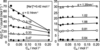Figure 2 Examples for the extrapolation to zero concentration for [Na+]* = 0.42 mol l−1. In the case of q > 0.33 nm−1, I(q)/CD became almost independent of CD.

In this paper, we found that the obtained P(q) can be described well with a core–shell cylinder model of infinite length: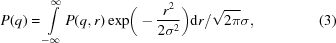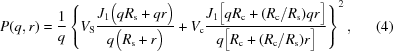where σ is the standard deviation, J1(x) is the first-order Bessel function, Rc and Rs are the core and total (core + shell) radii, and Vc and Vs are the parameters related to the electron density differences between the core and the shell, and the shell and the solvent (Pedersen, 1997). In equation (4), we assumed that the distributions of Rc and Rs are not independent for convenience.

### 4. Results and discussion

#### 4.1. Form factors

Panels A–E in Fig. 3present I(q)/CD at different CD for each [Na+]*. For all [Na+]*, with increasing CD the low-q intensities decreased and a shoulder appeared around q = 0.8–1.0 nm−1. This feature can be ascribed to concentration effects, indicating that inter-micellar diffraction becomes appreciable. It is interesting that the scattering profiles were drastically changed with increasing [Na+]*, especially for the low-q profiles. This feature may be ascribed to both micelle structural changes and the electron density changes of the solvent. For [Na+]* = 0.42, 0.75 and 1.00 mol l−1, extrapolation to CD = 0 could be carried out numerically and the resultant profiles [hereinafter denoted by I(q)C=0] are shown at the top of each panel. For [Na+]* = 1.25 and 1.50 mol l−1, we could not extrapolate the data because the intensities in the low-q region were too weak.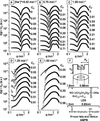Figure 3 Panels A–E: CD dependence of I(q)/CD and the P(q) profiles that were obtained by the extrapolation of CD = 0, and comparison with the theoretical calculation (solid lines) based on the core–shell cylinder model with Rc = 1.2 nm, Rs = 3.1 nm and Vc = −10. Panel F: the schematic illustration of the core–shell cylinder model and illustration of the definitions of the structural parameters; the perpendicular direction is the electron density. The chemical structures of LES and AMPB, and their sizes are also presented.

Fig. 4presents the cross-sectional plots of I(q)C=0 for [Na+]* = 0.42, 0.75 and 1.0 mol l−1. At q2 < 0.05 nm−2, the data points can be fitted by a straight line. This feature indicates that I(q)C=0 shows the asymptotic behaviour of I(q) ∼ 1/q in the low-q region which is expected for rodlike scattering objects. From the slope of the data, we calculated the cross-sectional radius of gyration (Rgc) by use of the following equation: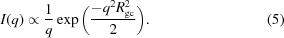The resultant values are presented at the right side of Fig. 4, showing that Rgc increased from 2.75 to 5.34 nm with increasing [Na+]*. For a uniform cylindrical particle with a radius of R, Rgc is given by R/21/2, which leads to the result that R increased from 3.9 to 7.5 nm. Interpretation of this result will be discussed later. According to previous studies that measured neutron scattering from wormlike micelles (Schurtenberger et al., 1991; Jerke et al., 1998), the exponent α in P(q) ∝ qα changes from 1 to 2 with decreasing q. This transition occurs at q × lp ≃ 1.9 (where lp is the persistence length of the wormlike micelles) (Schurtenberger et al., 1991). We did not observe such a transition. This is because our minimum q is too large to observe this change of α. Therefore, we can conclude that P(q) is controlled by distances over which the micelles are rodlike rather than flexible.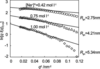Figure 4 Plots of ln[qI(q)] versus q2 (cross-sectional plot) at different [Na+]* and the linear fit to the low-q region which was used to determine Rgc.

To describe I(q)C=0, we adopted a core–shell cylinder model with infinite length [equation (4)]. Since our q range is much larger than 1/L and 1/lp, we considered that infinite cylinders are suitable. In equation (4), the electron density in the cylindrical micelles varies in a rectangular manner at the interface between the core and the shell, and between the shell and the solvent. The core and shell regions presumably consist mainly of the surfactant tails and head groups, respectively. To obtain the best-fit parameters, we have used two consecutive procedures. In the first step, we have used a homogeneous cylinder model (i.e. σ = 0) and obtained a set of the best-fit parameters of Rc, Rs, Vc and Vs by an iteration method. In the second step, the appropriate σ values were determined and then fine tuning of Rc, Rs, Vc and Vs was carried out. The parameters obtained are presented in Table 1and shown plotted against [Na+]* in Fig. 5. Here, the error of the estimation was within ±0.05 nm for both Rc and Rs. The solid lines in panels A–C (Fig. 3) show the calculated values of P(q) from equations (3)and (4)using the parameters in Table 1, compared with the experimental data of I(q)C=0. The calculated values agree with the experimental data very well.

 Table 1The obtained core–shell cylinder parameters used to fit the form factors
[Na+]* Rs (nm) Rc (nm) Vs Vc σ (nm)
0.42 3.0 1.2 14.1 −10 0.23
0.75 3.1 1.2 12.9 −10 0.23
1.00 3.1 1.2 12.0 −10 0.28
1.25 3.1 1.2 11.1 −10 0.25
1.50 3.1 1.2 10.2 −10 0.25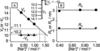Figure 5 [Na+]* dependence of the structural parameters and schematic comparison of Vs and Vc.

It is interesting that Rc and Rs do not depend on [Na+]*, when Rc = 1.2 nm and Rs = 3.1 nm for [Na+]* = 0.42, 0.75 and 1.00 mol l−1. The only structural parameter affected by [Na+]* was Vs, and the relation of Vs and [Na+]* can be fitted by a straight line. Since Rc, Rs and Vc are independent of [Na+]* and the linear relation between Vs and [Na+]* is held, we can predict the parameter sets for [Na+]* = 1.25 and 1.5 mol l−1, and the results are presented in Table 1. Based on these parameters, we calculated the theoretical P(q) for each and compared them with that of CD = 13 or 25 mmol l−1 for [Na+]* = 1.25 and 1.5 mol l−1 in panels D and E, respectively. Here the concentration effect can be considered negligibly small for these CD. For both cases, agreement between the theoretical prediction and experiment is good enough to conclude that the predicted parameters are reliable.

The values of Rs and Rc are found to be comparable with those of the headgroups and tails for LES and AMPB molecules (see Fig. 3for the size of the molecules), confirming that they form conventional cylindrical micelles. The unchanged Rs, Rc and Vc mean that the local molecular packing between the headgroups is not changed by [Na+]*. In fact, this conclusion is rather surprising because we expected that the reduced electrostatic repulsion might bring the headgroups close to each other, or at least the structural parameters might be changed corresponding to decreasing lifetime of the entanglement junction (see the inset of Fig. 1). With increasing [Na+]*, the scattering intensities in the low-q range dramatically drop as presented in Fig. 2,panels D and E. These changes are not owing to the structural changes of the cross section of the micelle, but should be ascribed to the electron density increment of the solvent, which decreases Vs, as schematically presented in the inset of Fig. 3.

Fig. 4showed that Rgc decreased with increasing [Na+]*. This change did not reflect the dimensional changes of the micelles, but the change of salt concentration of the solvent. With increasing [Na+]*, the electron density of the solvent becomes close to that of the core, as presented in the inset of Fig. 5. The origin of the contrast for SAXS from solutions is the electron density difference between the scattering objects and solvents. Therefore, the situation in which the core and solvent have a close electron density provides strong contrast to the shell. This is the reason that Rgc increased with increasing [Na+]*.

#### 4.2. Structure factors

Fig. 6presents S(q) obtained from equation (2)for [Na+]* = 0.75, 1.00, 1.25 and 1.50 mol l−1. For each case, with increasing CD the intensity of the first maximum increases and the peak position shifts to higher q, and the second one becomes appreciable. This feature can be considered as a sign of formation of a local order in the system and is a well known feature also observed in polyelectrolyte solutions. Using the Bragg equation, the mean distance between the micelles (dm) was determined for each CD and plotted against CD for all [Na+]* in Fig. 7. The dm value decreased with increasing CD. This means that the micelles are, on average, closer to each other with increasing CD. A similar shift of dm is observed at fixed CD and with increasing [Na+]*. In this case, the decrease of dm may be considered due to the weakening of the electrostatic repulsion as the screening is increased. Furthermore, the peak height (which is related to the order of a structure) seems to decrease with increasing [Na+]*. These two results suggest that there is repulsion present between the micelles and the origin of this repulsion is an electrostatic interaction, although the ionic strength is relatively large. When we plotted dm2 against CD, it seemed that the most linear relationship was obtained compared with other plots; this is consistent with dm being related to the inter-wormlike cylinder distance. We observe exactly the same behaviour as reported for polymer–electrolyte and salt free (or low-ionic strength) wormlike micelle solutions (Sommer et al., 2002).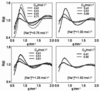Figure 6 CD dependence of the S*(q) profiles for [Na+]* = 0.75, 1.00, 1.25 and 1.50 mol l−1. The number attached to each profile is CD in mol l−1.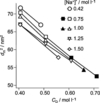Figure 7 CD dependence of dm for all [Na+]* samples. The filled marks indicate the presence of slow relaxations observed with rheological measurements.

Cannavacciuolo et al. (2002) carried out a Monte Carlo simulation on systems consisting of many charged wormlike micelles with excluded volume and electrostatic interactions and concluded that the scattering function becomes almost identical with that of uncharged system when the ionic strength is more than 0.1 mol l−1. This is because the electrostatic screening due to added salt is large enough to reduce repulsion between charged micelles. Their model was able to accurately reproduce the structural peak of the scattering function for charged micelles (Sommer et al., 2002). The CD dependence of dm in Fig. 7coincides with their calculation.

According to the rheological measurements, slow and distributed relaxations were observed at CD > 0.6 mol l−1 at [Na+]* = 0.75 and 1.00 mol l−1. In this region, the dm value becomes approximately twice that of Rs. This feature means that the micelles push each other and thus direct contact between them becomes possible. At CD > 0.7, we can expect that dm decreases further, which is consistent with the fact that liquid–liquid phase separation takes place at CD > 0.7.

### 5. Conclusions

SAXS data on LES/AMPB/water systems were systematically obtained as a function of [Na+]* and CD. The form factor was experimentally obtained by extrapolating I(q)/CD to CD = 0 and the resultant profiles were well fitted with a core–shell cylinder model for all [Na+]*. The parameters of Rc, Rs and Vc were 1.2 ± 0.05 nm, 3.1 ± 0.05 nm and −10 for all [Na+]*, indicating that the cross-sectional micelle structure does not depend on the salt concentration. S(q) was obtained by use of equation (2)and thus dm was obtained at the first maximum peak of S(q). The dm value decreased with increasing CD and [Na+]*. These features indicate that repulsion (presumably due to electrostatic interactions) between the micelles is still present at such high salt concentrations. The rheological measurements had shown presence of slow relaxations in the range of CD > 0.60 mol l−1 for [Na+]* = 1.0 and 0.75 mol l−1. In these ranges, dm became 2.5Rs.

### Acknowledgements

The present work was supported by Grant-in-Aid for Scientific Research (16350068 & 16655048) and the SAXS was carried out SPring-8 BL40B2 (2005B0150, 2005B0405, 2006A1510, 2006A1534).

### ReferencesJOURNAL OFAPPLIEDCRYSTALLOGRAPHY